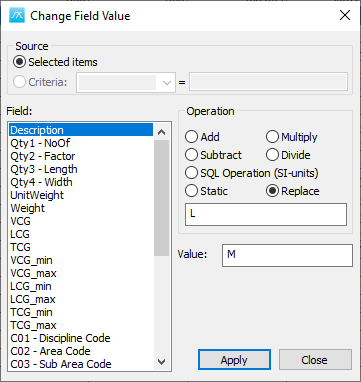﻿ ShipWeight Basics > The Item Window: Entering Weight Information > Change Field Value in Items Window

# Change Field Value in Item Window

From the Items menu in the Items Window, select Change Field Values… to open a pop up window called Change Field Value Window. Here you can perform 4 different types of operations on item values in the Item Window, on a single item or on a selection of multiple items.

The 4 different types of operations are:

1. Mathematical operations such as adding, subtracting, multiplying and dividing.
2. SQL Operation (SI-units).
3. Static value.
4. Find/replace.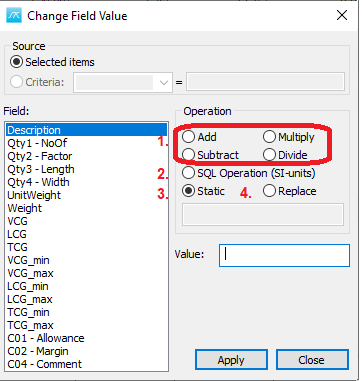Select the column or columns you want to carry out the operation on, specify type of operation and function value for the operation. When clicking Apply, the selected operation will be carried out on the marked weight items in the Item Window. Below the different operations are explained a bit more detailed.

# 1. Mathematical operations such as adding, subtracting, multiplying and dividing.

First select the columns you would like to do a mathematical operation on. Then open the Change Field Values Window by clicking the Items menu and selecting Change Field Values... .To perform a mathematical operation on a numerical field in the selected column(s) you need to select that field in the Field selection (1). Then select add, subtract, multiply or divide in the operation field (2) thereafter enter in the value you would like to add, subtract, multiply with or divide the selected field with in the Value field (3). When this is done click apply to execute the calculation. (4)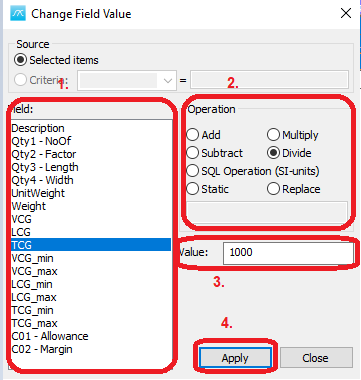Example: The QA-test discovered that TCG values were given in millimeters instead of meters for some items, correct the TCG values to be in meters.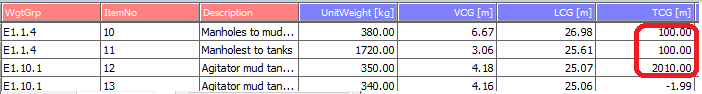1. Select the columns containing the TCG with unit given in mm.2. Open the Change Field Values... window.3. Set field to be TCG.

4. Select the Operation to be Divide.

5. Set the value to 1000.

6. Click Apply.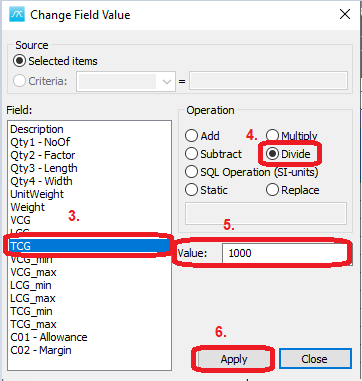Now the selected columns have values given in meters in stead of mm.# 2. SQL Operation (SI-units).

If you would like to calculate a value for a field to be respective to another field the SQL operation can be used.

Example: Calculate LCG_min = LCG- 2.

First set the Field to be LCG_min, then set the Operation to be SQL Operation (SI-units), thereafter write in the expression in the value field: LCG - 2. When you click Apply the operation is executed for any items marked.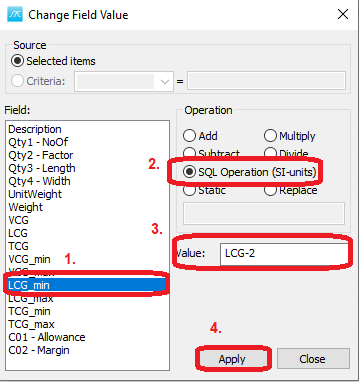# 3. Static value.

To set a static value for one or more column(s) use the static operation.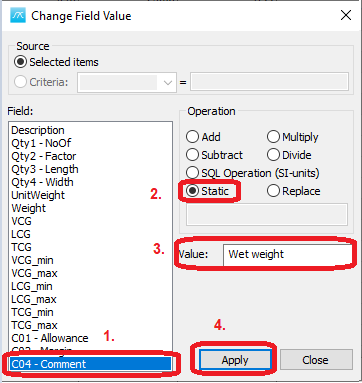# 4. Find/replace.

The last operation is a find replace. Select the Field you want to find/replace something in, set the Operation to Replace. Enter the text to be replaced in the box inside the Operation section, and then enter in the replacement in the value box.

See the picture below as a small example of how to look for the letter “L” in the “Description” field and replace all Ls with “M” for any items marked.